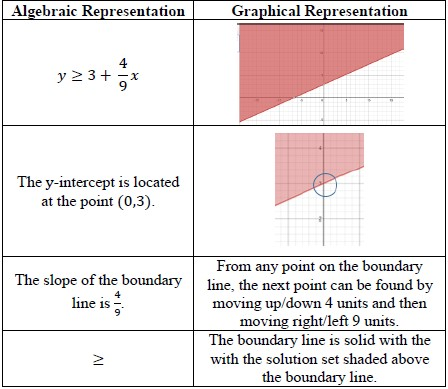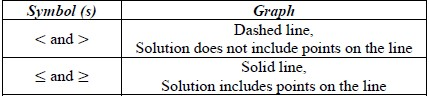# MA.912.AR.9.4Export Print
Graph the solution set of a system of two-variable linear inequalities.

### Clarifications

Clarification 1: Instruction includes cases where one variable has a coefficient of zero.

Clarification 2: Within the Algebra 1 course, the system is limited to two inequalities.

General Information
Subject Area: Mathematics (B.E.S.T.)
Strand: Algebraic Reasoning
Status: State Board Approved

## Benchmark Instructional Guide

• Inequality

### Vertical Alignment

Previous Benchmarks

Next Benchmarks

### Purpose and Instructional Strategies

In grade 8, students solved two-step linear inequalities and determined graphically whether a system of linear equations had one solution, no solution or infinitely many solutions. In Algebra I, students solve systems of linear inequalities by graphing the solution set. In later courses, students will solve problems involving linear programming.
• For students to have full understanding of systems, instruction includes MA.912.AR.9.4 and MA.912.AR.9.6. Equations and inequalities and their constraints are all related and the connections between them should be reinforced throughout the instruction.
• Instruction includes the use of linear inequalities in standard form, slope-intercept form and point-slope form. Include examples in which one variable has a coefficient of zero 17 such as $x$ < −$\frac{\text{17}}{\text{5}}$
• Instruction includes the connection to graphing solution sets of one-variable inequalities on a number line, recognizing whether the boundary line should be dotted (exclusive) or solid (inclusive). Additionally, have students use a test point to confirm which side of the line should be shaded (MTR.6.1).
• Students should recognize that the inequality symbol only directs where the line is shaded (above or below) for inequalities when in slope-intercept form. Students shading inequalities in other forms will need to use a test point to determine the correct half-plane to shade.
• The solution to a system of inequalities is the area where all the shading overlaps. If the areas do not overlap, it has no solution.
• Instruction includes determining whether the point of intersection of the boundary lines of the linear inequalities is within the solution set.
• For example, if either or both of the two boundary lines are dashed (< or >), then the point of intersection is not in the solution set.
• Instruction allows students to make connections between the algebraic and graphical representations of inequalities in two variables (MTR.2.1).

### Common Misconceptions or Errors

• Students may have difficulties making connections between graphic and algebraic representations of systems of inequalities.
• Students may confuse which points are in the solution set of a system that includes inequalities (including points on the lines in a system of inequalities).
• Students may shade the wrong half-plane or graph the incorrect boundary line (solid vs. dashed).

### Strategies to Support Tiered Instruction

• Instruction includes making the connection between the algebraic and graphical representations of a two-variable linear inequality and its key features.
• For example, teacher can provide a graphic organizer such as the one below.• Instruction includes using different colors or shapes to identify each of the solution sets of the linear inequalities.
• For example, a student can “shade” the solution of the first inequality by putting several triangles on its half-plane and can “shade” the solution of the second inequality by putting several rectangles on its half-plane. Where the triangles and rectangles overlap represent the solution set of the system of linear inequalities.
• Teacher creates connections to solving a system of linear inequalities to determining the solution set to a single two-variable linear inequality, building on students’ knowledge from MA.912.AR.2.8
• For example, a student can focus first on finding the solution set of one of the inequalities by graphing the boundary line and then choosing a test point to determine where to shade. Next, the student can focus on finding the solution set of the second inequality in the same way. Students should understand that where the two shaded regions overlap is the solution set of the system.
• Instruction includes using transparencies to lay the separately graphed inequalities on top of one another to visualize the solution set of the system.
• Teacher co-creates a graphic organizer to scaffold graphing an inequality and its solution. The steps can be repeated for each inequality.
• Example:• Part A. Graph the solution set to the system of inequalities:
$y$ > −$x$ + 4       $y$ < $\frac{\text{2}}{\text{5}}$$x$ + 2
• Part B. What is one point that is a solution to the system above?

• Devonte is throwing a party to watch the Stanley Cup Finals. He orders pizza that cost \$11 each and cartons of wings that cost \$9.99 each. With at least 34 people coming over, Devonte spends at least \$72.96 and orders a minimum of 7 pizzas and cartons of wings.
• Part A. Write a system of inequalities that describes this situation.
• Part B. Graph the solution set and determine a possible number of pizza and cartons of wings he ordered for his party.

### Instructional Items

Instructional Item 1
• Graph the solution set to the system of inequalities below.
$x$ ≥ 3        $\frac{\text{3}}{\text{5}}$$x$ + $y$ < −3

Instructional Item 2
• Graph the solution set to the system of inequalities below.
−$y$ − 3$x$ ≤ −1     2$y$ ≥ 8$x$ − 6

*The strategies, tasks and items included in the B1G-M are examples and should not be considered comprehensive.

## Related Courses

This benchmark is part of these courses.
1200310: Algebra 1 (Specifically in versions: 2014 - 2015, 2015 - 2022, 2022 and beyond (current))
1200320: Algebra 1 Honors (Specifically in versions: 2014 - 2015, 2015 - 2022, 2022 and beyond (current))
1200370: Algebra 1-A (Specifically in versions: 2014 - 2015, 2015 - 2022, 2022 and beyond (current))
1200400: Foundational Skills in Mathematics 9-12 (Specifically in versions: 2014 - 2015, 2015 - 2022, 2022 and beyond (current))
7912080: Access Algebra 1A (Specifically in versions: 2014 - 2015, 2015 - 2018, 2018 - 2019, 2019 - 2022, 2022 and beyond (current))
1200315: Algebra 1 for Credit Recovery (Specifically in versions: 2014 - 2015, 2015 - 2022, 2022 and beyond (current))
1200375: Algebra 1-A for Credit Recovery (Specifically in versions: 2014 - 2015, 2015 - 2022, 2022 and beyond (current))
7912075: Access Algebra 1 (Specifically in versions: 2014 - 2015, 2015 - 2018, 2018 - 2019, 2019 - 2022, 2022 and beyond (current))
1200710: Mathematics for College Algebra (Specifically in versions: 2022 and beyond (current))

## Related Access Points

Alternate version of this benchmark for students with significant cognitive disabilities.
MA.912.AR.9.AP.4: Select the graph of the solution set of a system of two-variable linear inequalities.

## Related Resources

Vetted resources educators can use to teach the concepts and skills in this benchmark.

## Formative Assessments

Graph a System of Inequalities:

Students are asked to graph a system of two linear inequalities.

Type: Formative Assessment

Which Graph?:

Students are asked to select the correct graph of the solution region of a given system of two linear inequalities.

Type: Formative Assessment

## Lesson Plan

This lesson allows students to graph systems of linear inequalities with two variables and view three possible types of solutions.

Type: Lesson Plan

## MFAS Formative Assessments

Graph a System of Inequalities:

Students are asked to graph a system of two linear inequalities.

Which Graph?:

Students are asked to select the correct graph of the solution region of a given system of two linear inequalities.

## Student Resources

Vetted resources students can use to learn the concepts and skills in this benchmark.

## Parent Resources

Vetted resources caregivers can use to help students learn the concepts and skills in this benchmark.